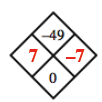### Home > MC2 > Chapter 5 > Lesson 5.2.4 > Problem5-81

5-81.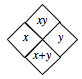Copy and complete each of the Diamond Problems below. The pattern used in the Diamond Problems is shown below. Homework Help ✎

 a.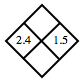b.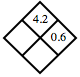c.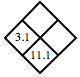d.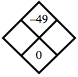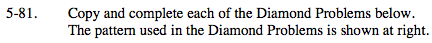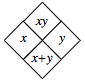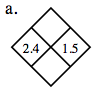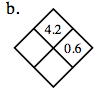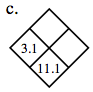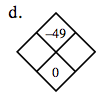What are the product and sum of 2.4 and 1.5?

? (0.6) = 4.2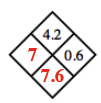3.1 + ? = 11.1

Which two numbers have a product of -49 and a sum of 0?

Since the sum is 0, one number must be negative and one must be positive, but both numbers must have the same magnitude.